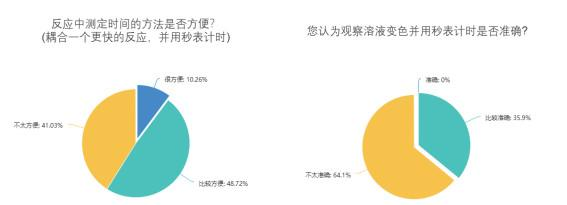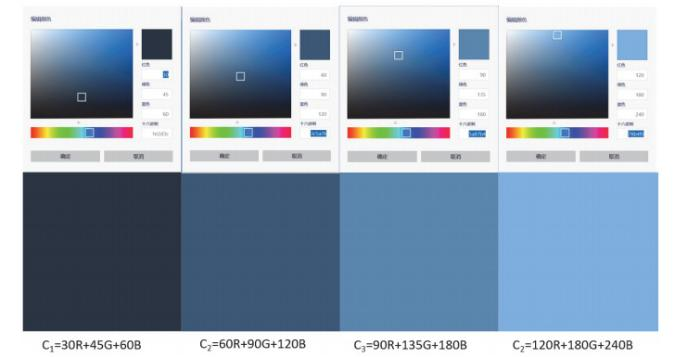## Determination of Kinetic Constant of Methyl Violet with Sodium Hydroxide Using Smart Phone

Zeng Yifan, Qin Xiao, Wang Yue, Li Zhao,

§2018级本科生

 基金资助: 国家基础科学人才培养基金项目.  J1310024Abstract

The chemical reaction rate is the basic concept of reaction kinetics, and chemical kinetic experiments to determine rate laws are common in college chemistry laboratory courses. However, there are many shortcomings in this experiment, with the large consumption of reagents as one of them. Starting from the concept of green chemistry, we propose to combine the color theory with chemical knowledge, so as to save the reagents used in the experiment and improve the students' interest in the experiment. In this paper, we first conducted a survey to investigate the subjective evaluation on classical kinetics experiments and the willingness to do virtual chemical experiments with smart phones. After analyzing the feasibility of the method in theory, we shot a short video of the reaction of methyl violet with sodium hydroxide, and determination of the kinetic constant using colorimetric analysis. The method combines the complementary color theory and Lamber-Beer law, and a dynamic experiment can be carried out by using smart phone and commonly used software only.

Keywords： Kinetic constant ; Colorimetric analysis ; Green chemistry ; Practice research

Zeng Yifan. Determination of Kinetic Constant of Methyl Violet with Sodium Hydroxide Using Smart Phone. University Chemistry[J], 2020, 35(6): 54-62 doi:10.3866/PKU.DXHX202002001

 试剂种类和浓度 试剂用量/mL 浓度对化学反应速率的影响实验 温度对化学反应速率的影响实验 完整实验 0.2 mol·L-1 KI溶液 75 15 90 0.2%淀粉溶液 20 6 26 0.010 mol·L-1 Na2S2O3溶液 40 12 52 0.20 mol·L-1 KNO3溶液 25 15 40 0.20 mol·L-1 (NH4)2SO4溶液 25 0 25 0.20 mol·L-1 (NH4)2S2O8溶液 75 30 105 各类试剂总和 260 78 338

### 图1### 图2### 图3### 图4## 2 色度分析测定浓度的探究

$\mathrm{C}=r \mathrm{R}+g \mathrm{G}+b \mathrm{B}$

### 图5$\begin{array}{l}I=k_{r} \times r \end{array}$

$I=k_{g} \times g$

$I=k_{b} \times b$

$A=\lg \frac{I_{0}}{I_{\mathrm{t}}}=\lg \frac{r_{0}}{r_{\mathrm{t}}}=\varepsilon b c$

$A=\lg \frac{I_{0}}{I_{\mathrm{t}}}=\lg \frac{g_{0}}{g_{\mathrm{t}}}=\varepsilon b c$

$A=\lg \frac{I_{0}}{I_{\mathrm{t}}}=\lg \frac{b_{0}}{b_{\mathrm{t}}}=\varepsilon b c$

### 3.1 实验原理

NaOH与甲基紫的反应方程式如下：

$\mathrm{CV}^{+}+\mathrm{OH}^{-} \rightarrow \mathrm{CVOH}$

$\begin{array}{l}-\frac{\mathrm{d}\left[\mathrm{CV}^{+}\right]}{\mathrm{d} t}=k\left[\mathrm{CV}^{+}\right]\left[\mathrm{OH}^{-}\right] \end{array}$

$-\frac{\mathrm{d}\left[\mathrm{CV}^{+}\right]}{\mathrm{d} t}=k^{\prime}\left[\mathrm{CV}^{+}\right]$

$\ln \left[\mathrm{CV}^{+}\right]=-k^{\prime} t+\left[\mathrm{CV}^{+}\right]_{0}$

$k=\frac{2 k^{\prime}}{c_{\mathrm{NaOH}}}$

### 3.2 实验试剂

#### 3.3.2 标准溶液配制

 编号 甲基紫加入体积/mL 定容体积/mL 甲基紫浓度/(μmol·L-1) 1 0.00 50.0 0.00 2 5.0 50.0 10.0 3 10.0 50.0 20.0 4 15.0 50.0 30.0 5 20.0 50.0 40.0 6 25.0 50.0 50.0

#### 3.3.3 实验录像

1)将上述标准溶液分别加入1–6号比色皿(图6)。

### 图6(a) t = 10 s；(b) t = 35 s；(c) t = 85 s；(d) t = 145 s

2)向标注为S的比色皿(图6)中加入50 μmol·L-1的甲基紫2 mL。

3)将上述所有比色皿放置在白色A4纸前，手机与中心比色皿保持30 cm以上的距离。

4)将0.2 mol·L-1的NaOH溶液2 mL加入比色皿S中，手机锁定白平衡和聚焦，开始录像3 min (到紫色大部分明显褪去即可)。反应均在室温下进行。

### 4.1 r, g, b值的读取和工作曲线的绘制

1)任选一个时间点的视频截图，在该视频截图中的每个比色皿内各取3点，读取R, G, B三个分量的数值，取平均值作为该份溶液的色度值。注意应在比色皿的同一高度进行读数。

2)按照式(5)–式(7)计算A值。

3)将求得的A分别对浓度作图，选择斜率最大的分量作为工作曲线来测定甲基紫的浓度。

### 4.2 反应速率实验曲线绘制

1)从视频时间0 s开始，每5 s截取一张图片，对装有待测样的比色皿S用上述3.1节中的方法确定吸光度，通过工作曲线进而确定浓度。

2)以浓度对时间作图，得浓度–时间关系图，其中时间t以加入NaOH溶液的时间为计时零点。

## 5 实验结果与讨论

### 图7### 图8### 图9### 6.1 实验方案对比

$\bar{v}=\frac{\Delta\left[\mathrm{S}_{2} \mathrm{O}_{8}^{2-}\right]}{\Delta t} \approx K\left[\mathrm{S}_{2} \mathrm{O}_{8}^{2-}\right]^{m}\left[\mathrm{I}^{-}\right]^{n}$

## 参考文献 原文顺序 文献年度倒序 文中引用次数倒序 被引期刊影响因子

Kehoe E. ; Penn R. L. J. Chem. Educ. 2013, 90 (9), 1191.

Campos A. R. ; Knutson C. M. ; Knutson T. R. ; Mozzetti A. R. ; Haynes C. L. ; Penn R. L. J. Chem. Educ. 2015, 93 (2), 318.

Knutson T. R. ; Knutson C. M. ; Mozzetti A. R. ; Campos A. R. ; Haynes C. L. ; Penn R. L. J. Chem. Educ. 2015, 92 (10), 1692.

/

 〈〉